# Class 12 Maths NCERT Solutions for Chapter 12 Linear Programming Miscellaneous Exercise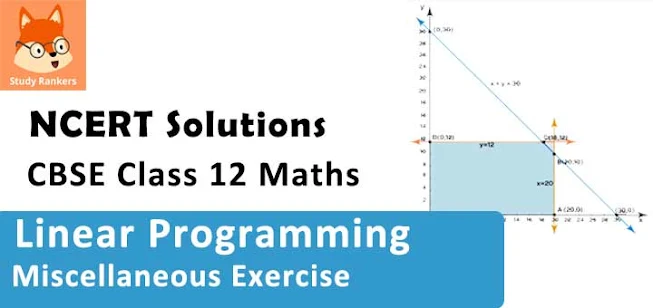### Linear Programming Miscellaneous Exercise Solutions

1. Refer to Example 9. How many packets of each food should be used to maximize the amount of vitamin A in the diet? What is the maximum amount of vitamin A in the diet?

Solution

Let the diet contain and y packets of foods P and Q respectively. Therefore,
x ≥ 0 and y ≥ 0
The mathematical formulation of the given problem is as follows.
Maximize = 6+ 3y …(1)
subject to the constraints,
4x + y ≥ 80 ...(2)
x + 5y ≥ 115 ...(3)
3x + 2y ≤ 150 ...(4)
x, y ≥ 0 ...(5)
The feasible region determined by the system of constraints is as follows.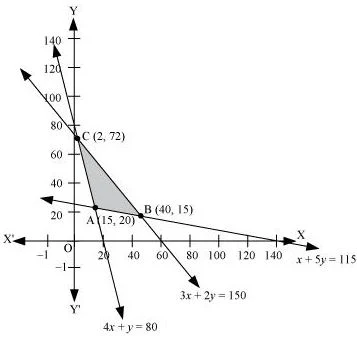The corner points of the feasible region are A (15, 20), B (40, 15), and C (2, 72).
The values of at these corner points are as follows.

 Corner point z = 6x + 3y A(15, 20) 150 B(40, 15) 285 → Maximum C(2, 72) 228

Thus, the maximum value of z is 285 at (40, 15).
Therefore, to maximize the amount of vitamin A in the diet, 40 packets of food P and 15 packets of food Q should be used. The maximum amount of vitamin A in the diet is 285 units.

2. A farmer mixes two brands P and Q of cattle feed. Brand P, costing Rs 250 per bag contains 3 units of nutritional element A, 2.5 units of element B and 2 units of element C. Brand Q costing Rs 200 per bag contains 1.5 units of nutritional elements A, 11.25 units of element B, and 3 units of element C. The minimum requirements of nutrients A, B and C are 18 units, 45 units and 24 units respectively. Determine the number of bags of each brand which should be mixed in order to produce a mixture having a minimum cost per bag? What is the minimum cost of the mixture per bag?

Solution

Let the farmer mix x bags of brand P and y bags of brand Q.
The given information can be compiled in a table as follows.

 Vitamin A (units/bag) Vitamin B (units/bag) Vitamin C (units/bag) Cost (Rs/bag) Food P 3 2.5 2 250 Food Q 1.5 11.25 3 200 Requirement (units/bag) 18 45 24

The given problem can be formulated as follows.
Minimize z = 250x + 200y …(1)
subject to the constraints,
3x + 1.5y ≥ 18 …(2)
2.5x + 11.25y ≥ 45 ...(3)
2x + 3y ≥ 24 …(4)
x, y ≥ 0 …(5)
The feasible region determined by the system of constraints is as follows.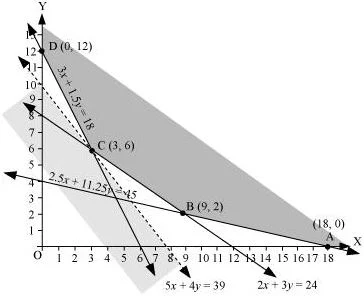The corner points of the feasible region are A (18, 0), B (9, 2), C (3, 6), and D (0, 12).

The values of z at these corner points are as follows.

 Corner point z = 250x + 200y A (18, 0) 4500 B (9, 2) 2650 C (3, 6) 1950 → Minimum D (0, 12) 2400

As the feasible region is unbounded, therefore, 1950 may or may not be the minimum value of z.
For this, we draw a graph of the inequality, 250x + 200y < 1950 or 5x + 4y < 39, and check whether the resulting half plane has points in common with the feasible region or not.
It can be seen that the feasible region has no common point with 5x + 4y < 39
Therefore, the minimum value of z is 1950 at (3, 6).
Thus, 3 bags of brand P and 6 bags of brand Q should be used in the mixture to minimize the cost to Rs 1950.

3. A dietician wishes to mix together two kinds of food X and Y in such a way that the mixture contains at least 10 units of vitamin A, 12 units of vitamin B and 8 units of vitamin C. The vitamin content of one kg food is given below:

 Food Vitamin A Vitamin B Vitamin C X 1 2 3 Y 2 2 1
One kg of food X costs Rs 16 and one kg of food Y costs Rs 20. Find the least cost of the mixture which will produce the required diet?
Solution
Let the mixture contain x kg of food X and y kg of food Y.
The mathematical formulation of the given problem is as follows.
Minimize z = 16x + 20y … (1)
subject to the constraints,
x + 2y ≥ 10 ...(2)
x + y  ≥ 6 ...(3)
3x + y ≥ 8 ...(4)
x, y ≥  0 ...(5)
The feasible region determined by the system of constraints is as follows.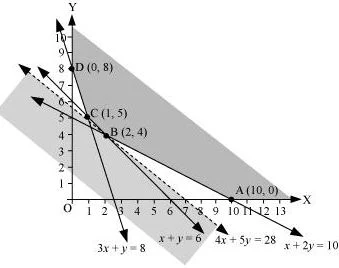The corner points of the feasible region are A (10, 0), B (2, 4), C (1, 5), and D (0, 8).
The values of z at these corner points are as follows.

 Corner point z = 16x + 20y A (10, 0) 160 B (2, 4) 112 → Minimum C (1, 5) 116 D (0, 8) 160

As the feasible region is unbounded, therefore, 112 may or may not be the minimum value of z.
For this, we draw a graph of the inequality, 16x + 20y < 112 or 4x + 5y < 28, and check whether the resulting half plane has points in common with the feasible region or not.
It can be seen that the feasible region has no common point with 4x + 5y < 28
Therefore, the minimum value of z is 112 at (2, 4).
Thus, the mixture should contain 2 kg of food X and 4 kg of food Y. The minimum cost of the mixture is Rs 112.

4. A manufacturer makes two types of toys A and B. Three machines are needed for this purpose and the time (in minutes) required for each toy on the machines is given below:

 Type of toys Machines I II III A 12 18 6 B 6 0 9

Each machine is available for a maximum of 6 hours per day. If the profit on each toy of type A is Rs 7.50 and that on each toy of type B is Rs 5, show that 15 toys of type A and 30 of type B should be manufactured in a day to get maximum profit.

Solution

Let x and y toys of type A and type B respectively be manufactured in a day.
The given problem can be formulated as follows.
Maximize z = 7.5x + 5y  …(1)
subject to the constraints,
2x + y ≤ 60 …(2)
x ≤ 20 …(3)
2x + 3y ≤ 120 …(4)
x, y ≥ 0 …(5)
The feasible region determined by the constraints is as follows.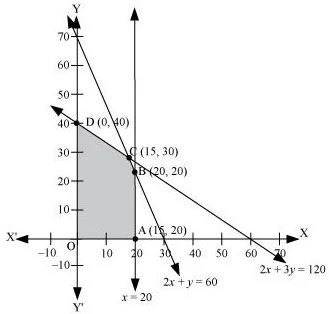The corner points of the feasible region are A (20, 0), B (20, 20), C (15, 30), and D (0, 40).
The values of z at these corner points are as follows.

 Corner point Z = 7.5x + 5y A(20, 0) 150 B(20, 20) 250 C(15, 30) 262.5 → Maximum O(0, 40) 200

The maximum value of z is 262.5 at (15, 30).
Thus, the manufacturer should manufacture 15 toys of type A and 30 toys of type B to maximize the profit.

5. An aeroplane can carry a maximum of 200 passengers. A profit of Rs 1000 is made on each executive class ticket and a profit of Rs 600 is made on each economy class ticket. The airline reserves at least 20 seats for executive class. However, at least 4 times as many passengers prefer to travel by economy class than by the executive class. Determine how many tickets of each type must be sold in order to maximize the profit for the airline. What is the maximum profit?

Solution

Let the airline sell tickets of executive class and y tickets of economy class.
The mathematical formulation of the given problem is as follows.
Maximize z = 1000+ 600y …(1)
subject to the constraints,
x + y ≤ 200 …(2)
x ≥ 20 …(3)
y – 4x ≥ 0 …(4)
x, y ≥ 0 …(5)
The feasible region determined by the constraints is as follows.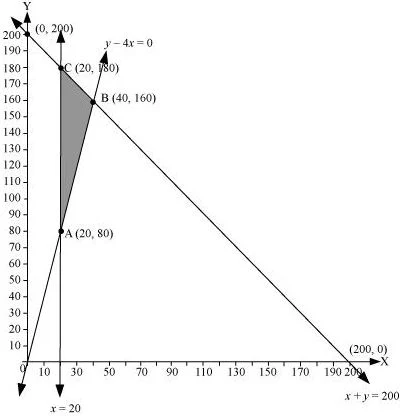The corner points of the feasible region are A (20, 80), B (40, 160), and C (20, 180).
The values of z at these corner points are as follows.

 Corner point z = 1000x + 600y A (20, 80) 68000 B (40, 160) 136000 → Maximum C (20, 180) 128000

The maximum value of z is 136000 at (40, 160).
Thus, 40 tickets of executive class and 160 tickets of economy class should be sold to maximize the profit and the maximum profit is Rs 136000.

6. Two godowns A and B have grain capacity of 100 quintals and 50 quintals respectively. They supply to 3 ration shops, D, E and F whose requirements are 60, 50 and 40 quintals respectively. The cost of transportation per quintal from the godowns to the shops are given in the following table:

 Transportation cost per quintal (in Rs) From/To A B D E F 6 3 2.50 4 2 3

How should the supplies be transported in order that the transportation cost is minimum? What is the minimum cost?

Solution

Let godown A supply x and y quintals of grain to the shops D and E respectively. Then, (100 − x − y) will be supplied to shop F.
The requirement at shop D is 60 quintals since x quintals are transported from godown A. Therefore, the remaining (60 −x) quintals will be transported from godown B.
Similarly, (50 − y) quintals and 40 − (100 − x − y) = (x + y − 60) quintals will be transported from godown B to shop E and F respectively.
The given problem can be represented diagrammatically as follows.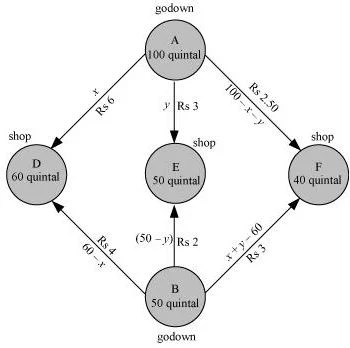x  ≥ 0, y ≥ 0, and  100 - x - y ≥ 0
⇒ x ≥ 0, y≥ 0, and x + y ≤ 100
60 - x ≥ 0, 50 - y ≥ 0, and x + y - 60 ≥ 0
⇒ x ≤ 60, y ≤ 50, and  x + y ≥ 60
Total transportation cost z is given by,
z = 6x + 3y +2.5(100 - x - y) + 4(60 - x) + 2(50 - y) + 3(x + y - 60)
= 6x + 3y + 250 - 2.5x - 2.5y + 240 - 4x + 100 - 2y + 3x + 3y - 180
= 2.5x + 1.5y + 410
The given problem can be formulated as
Minimize z = 2.5x + 1.5y + 410 …(1)
subject to the constraints,
x + y ≤ 100 ...(2)
x ≤ 60 ...(3)
y ≤ 50 ...(4)
x + y ≥ 60 ...(5)
x, y ≥ 0  ...(6)
The feasible region determined by the system of constraints is as follows.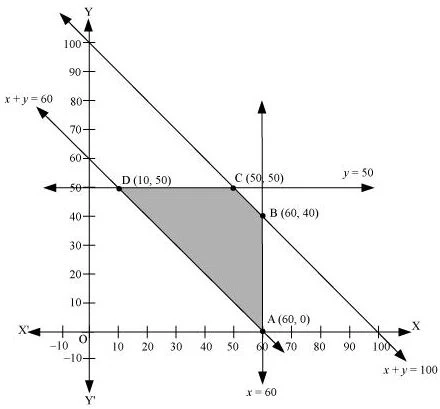The corner points are A(60, 0), B(60, 40), C(50, 50) and D(10, 50).
The values of z at these corner points are as follows.

 Corner point z = 2.5x + 1.5y + 410 A (60, 0) 560 B (60, 40) 620 C (50, 50) 610 D (10, 50) 510 → Minimum

The minimum value of z is 510 at (10, 50).
Thus, the amount of grain transported from A to D, E, and F is 10 quintals, 50 quintals, and 40 quintals respectively and from B to D, E, and F is 50 quintals, 0 quintals, and 0 quintals respectively.
The minimum cost is Rs 510.

7. An oil company has two depots A and B with capacities of 7000 L and 4000 L respectively. The company is to supply oil to three petrol pumps, D, E and F whose requirements are 4500L, 3000L and 3500L respectively. The distance (in km) between the depots and the petrol pumps is given in the following table:

 Distance in (km) From/To A B D E F 7 6 3 3 4 2

Assuming that the transportation cost of 10 litres of oil is Re 1 per km, how should the delivery be scheduled in order that the transportation cost is minimum? What is the minimum cost?

Solution

Let x and y litres of oil be supplied from A to the petrol pumps, D and E. Then, (7000 − x − y) will be supplied from A to petrol pump F.
The requirement at petrol pump D is 4500 L. Since x L are transported from depot A, the remaining (4500 −x) L will be transported from petrol pump B.
Similarly, (3000 − y) L and 3500 − (7000 − x − y) = (x + y − 3500) L will be transported from depot B to petrol pump E and F respectively.
The given problem can be represented diagrammatically as follows.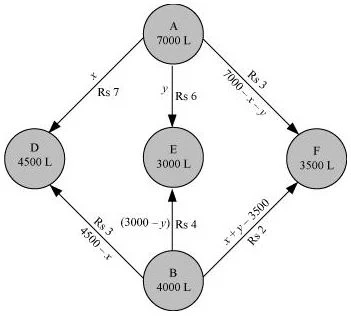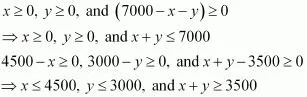Cost of transporting 10 L of  petrol = Re 1
Cost of transporting 1 of petrol = Rs 1/10
Therefore, total transportation cost is given by,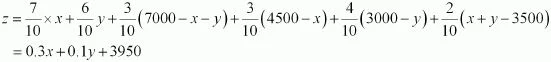The problem can be formulated as follows.
Minimize z = 0.3x + 0.1y + 3950 ...(1)
Subject to the constraints,
x + y ≤ 7000 ...(2)
x ≤ 4500 ...(3)
y ≤ 3000 ...(4)
x + y ≥   3500 ...(5)
x, y ≥ 0 ...(6)
The feasible region determined by the constraints is as follows .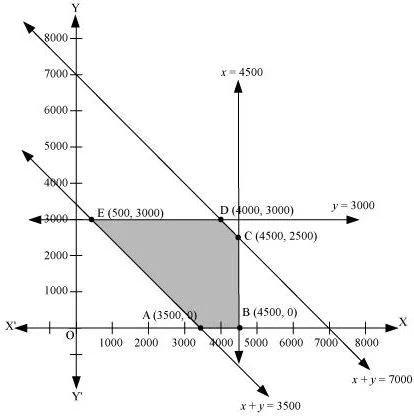The corner points of the feasible region are A (3500, 0), B (4500, 0), C (4500, 2500), D (4000, 3000), and E (500, 3000).
The values of z at these corner points are as follows.

 Corner point z = 0.3x + 0.1y + 3950 A (3500, 0) 5000 B (4500, 0) 5300 C (4500, 2500) 5550 D (4000, 3000) 5450 E (500, 3000) 4400 → Minimum

The minimum value of z is 4400 at (500, 3000).
Thus, the oil supplied from depot A is 500 L, 3000 L, and 3500 L and from depot B is 4000 L, 0 L, and 0 L to petrol pumps D, E, and F respectively.
The minimum transportation cost is Rs 4400.

8. A fruit grower can use two types of fertilizer in his garden, brand P and brand Q. The amounts (in kg) of nitrogen, phosphoric acid, potash, and chlorine in a bag of each brand are given in the table. Tests indicate that the garden needs at least 240 kg of phosphoric acid at least 270 kg of potash and at most 310 kg of chlorine.
If the grower wants to minimize the amount of nitrogen added to the garden, how many bags of each brand should be used? What is the minimum amount of nitrogen added in the garden?

 kg per bag Brand P Brand Q Nitrogen Phosphoric acid Potash Chlorine 3 1 3 1.5 3.5 2 1.5 2

Solution

Let the fruit grower use x bags of brand P and y bags of brand Q.
The problem can be formulated as follows.
Minimize z = 3x + 3.5y  …(1)
subject to the constraints,
x + 2y ≥ 240  ...(2)
x + 0.5y ≥ 90  ...(3)
1.5x + 2y ≤ 310  ...(4)
x, y ≥ 0  ...(5)
The feasible region determined by the system of constraints is as follows.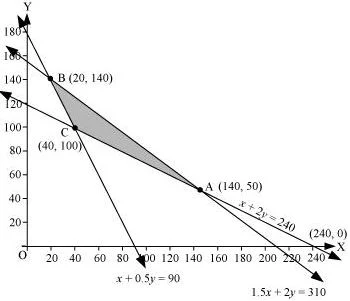The corner points are A (240, 0), B (140, 50), and C (20, 140).
The values of z at these corner points are as follows.

 Corner point z = 3x + 3.5y A (140, 50) 595 B (20, 140) 550 C (40, 100) 470 → Minimum

The maximum value of z is 470 at (40, 100).
Thus, 40 bags of brand P and 100 bags of brand Q should be added to the garden to minimize the amount of nitrogen.
The minimum amount of nitrogen added to the garden is 470 kg.

9. Refer to question 8. If the grower wants to maximize the amount of nitrogen added to the garden, how many bags of each brand should be added? What is the maximum amount of nitrogen added?

Solution

Let the fruit grower use x bags of brand P and y bags of brand Q.
The problem can be formulated as follows.
Maximize z = 3x + 3.5y …(1)
subject to the constraints,
x + 2y ≥ 240  ...(2)
x + 0.5y ≥ 90 ...(3)
1.5x + 2y ≤ 310  ...(4)
x, y ≥ 0  ...(5)
The feasible region determined by the system of constraints is as follows.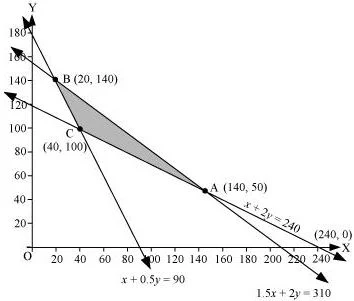The corner points are A (140, 50), B (20, 140), and C (40, 100).
The values of z at these corner points are as follows.

 Corner point z = 3x + 3.5y A (140, 50) 595 → Maximum B (20, 140) 550 C (40, 100) 470

The maximum value of z is 595 at (140, 50).
Thus, 140 bags of brand P and 50 bags of brand Q should be used to maximize the amount of nitrogen.
The maximum amount of nitrogen added to the garden is 595 kg.

10. A toy company manufactures two types of dolls, A and B. Market tests and available resources have indicated that the combined production level should not exceed 1200 dolls per week and the demand for dolls of type B is at most half of that for dolls of type A. Further, the production level of dolls of type A can exceed three times the production of dolls of other type by at most 600 units. If the company makes profit of Rs 12 and Rs 16 per doll respectively on dolls A and B, how many of each should be produced weekly in order to maximize the profit?

Solution

Let x and y be the number of dolls of type A and B respectively that are produced per week.
The given problem can be formulated as follows.
Maximize z = 12x + 16y …(1)
subject to the constraints,
x + y ≤ 1200 ...(2)
y ≤ x/2 ⇒ x ≥ 2y  ...(3)
x - 3y ≤ 600  ...(4)
x, y ≥ 0  ...(5)
The feasible region determined by the system of constraints is as follows.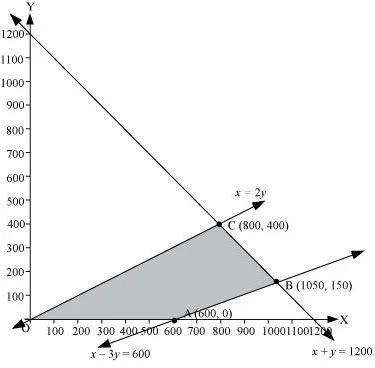The corner points are A (600, 0), B (1050, 150), and C (800, 400).
The values of z at these corner points are as follows.

 Corner point z = 12x + 16y A (600, 0) 7200 B (1050, 150) 15000 C (800, 400) 16000 → Maximum

The maximum value of z is 16000 at (800, 400).
Thus, 800 and 400 dolls of type A and type B should be produced respectively to get the maximum profit of Rs 16000.